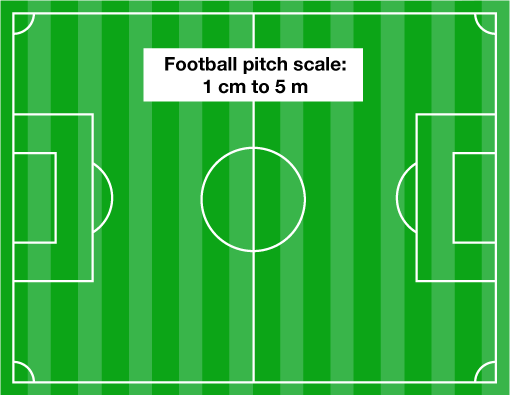Everyday maths 2 (Wales)

Start this free course now. Just create an account and sign in. Enrol and complete the course for a free statement of participation or digital badge if available.

# 5 Scale drawings and plans

If you completed Everyday maths 1, you will be familiar with the idea of scale. Scales are found on drawings, plans and maps and they are often written with the units indicated. Let’s look at an example.

A football pitch is drawn to the scale of 1 cm to 5 m.Figure 50 A football pitch drawn at 1 cm to 5 m

This means that every 1 cm measured on the plan is 5 m in real life.

If the plan is drawn with the length being 18 cm and the width being 9 cm, what are the dimensions of the football pitch in real life?

Write down the scale first:

• 1 cm to 5 m

You know the drawing dimensions so you need to work with these one at a time. Let’s start with the length of 18 cm:

• If the scale is 1 cm to 5 m then

•                       18 cm = ? m.

• If you have been given the drawing measurement and need to know the real life measurement, you multiply:

•      18 × 5 = 90 m

so the length is 90 m.

Note: If you have been given the actual measurement and need to find the drawing measurement you would need to divide.

Now you can work out the width measurement:

• If the scale is 1 cm to 5 m then

•                       9 cm = ? m.

• Again, you need to multiply:

•      9 × 5 = 45 m

so the width is 45 m.

A lot of scales are written differently, without the units indicated. The scale of 1 cm to 5 m could also be written as 1:500.

This is the scale expressed as a ratio and it is independent of any units. A scale of 1:500 means that the actual real-life measurements are 500 times greater than those on the plan or map. This means that it does not matter whether you take the measurements on the plan in millimetres (mm), centimetres (cm) or metres (m) – the measurements will be 500 times as much in real life.

To write a scale as a ratio, you often have to convert. Let’s look at the football pitch example again:

• 1 cm to 5 m

At the moment, the units of the scale are different. The plan side is given in centimetres (cm) and the real-life side is given in metres (m).

To express this as a ratio, you need to convert both sides to the same units. It is usually easiest to convert the real-life side of the scale into the same unit as the drawing side, so in this case it is easiest to convert 5 m into cm:

• 5 × 100 = 500 cm

So you can now write the scale as a ratio:

• 1:500

It is standard to try to write the ratio in the simplest form possible, ideally with a single unit (a ‘1’) on the drawing side of the ratio. This will make any calculations you do using the scale easier.

Now have a go a converting scales to ratios.

## Activity 9: Writing a scale as a ratio

Rewriting these scales as a ratio in their simplest form:

1.      1 cm to 2 m
2.      2 cm to 5 m
3.      10 mm to 20 m
4.      1 cm to 1 km
5.      5 cm to 2 km

1. It is easiest to change the 2 m into cm:

• 2 × 100 = 200 cm so the scale expressed as a ratio would be 1:200.
2. It is easiest to change the 5 m into cm:

• 5 × 100 = 500 cm so the scale expressed as a ratio could be written as:
•      2:500

However, we usually try to get the drawing side of the ratio down to a single unit (1) to make calculations easier. Therefore, you need to simplify the ratio. To do this here, divide both sides by 2:

• 2 ÷ 2 = 1
• 500 ÷ 2 = 250 so the scale can be written as:
•      1:250
3. It is easiest to change the 20 m into mm. It might be easiest to do this in stages:

• Convert to cm first –

1 m = 100 cm so 20 × 100 = 2000 cm

• Now convert to mm –

1 cm = 10 mm so 2000 × 10 = 20 000 mm

• This makes the scale:

•      10:20 000

• This can be simplified by dividing both sides by 10 to get:

•      1:2000

4. Change the 1 km into cm. Again, this will be easiest to do in stages:

• Convert to m first –

1 km = 1000 m so 1 × 1000 = 1000 m

• Now convert to cm –

1 m = 100 cm so 1000 × 100 = 100 000 cm

• This means the scale should be written as:

•      1:100 000

5. Change the 2 km into cm. In stages this can be done as follows:
• Convert to m first –

1 km = 1000 m so 2 × 1000 = 2000 m

• Now convert to cm –

1 m = 100 cm so 2000 × 100 = 200 000 cm

• This makes the scale:

•      5:200 000

• This can be simplified by dividing both sides by 5 to get:

•      1:40 000

Now you will look at using ratio scales to work out measurements.#### Find out more

*Eligibility rules apply for financial support.